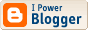Completed Script

As the comment under the description indicates, this script only has applicability in limited situations, but it works for what I'm doing right now and the result is spectacular. I'll revisit this later and make it work for multi-line cells, but don't hold your breath if you need it urgently.

Warning: This Script Has a Serious Problem; see next post.

//DESCRIPTION: Table Space Equalizer

/*
Expects a multi-column selection within a table. Adjusts widths of selected
columns so that space after the longest entry in each column is equalized and
the overall width of the table is unchanged.
*/

if ((app.documents.length == 0) || (app.selection.length == 0)) { exit() }

aSel = app.selection;

try {
theCols = {start: aSel.cells.name.split(":"),end: aSel.cells[-1].name.split(":")};
theRows = {start: aSel.cells.name.split(":"),end: aSel.cells[-1].name.split(":")};
theTable = aSel.parent;
} catch (e) {
alert("Selection needs to be in a table, and rectangular"), exit();
}

// For each column, calculate the min spare space:
minSpaces = new Array();
for (c = theCols.start; theCols.end >= c; c++) {
minSpace = Infinity;
for (r = theRows.start; theRows.end >= r; r++) {
minSpace = Math.min(minSpace, space(getCell(theTable, c, r,)));
}
minSpaces.push(minSpace);
}
totSpace = 0;
for (j = 0; minSpaces.length > j; j++) {
totSpace = totSpace + minSpaces[j]
}
newSpace = totSpace/minSpaces.length;
for (c = theCols.start; theCols.end >= c; c++) {
theTable.columns[c].width = theTable.columns[c].width + newSpace - minSpaces[c - theCols.start];
}

function getCell(table, c, r) {
return table.cells.item(c + ":" + r);
}

function space(theCell) {
// This version assumes that the cell is left aligned and there's only one line
var left = theCell.texts.insertionPoints.horizontalOffset;
theCell.texts.justification = Justification.rightAlign;
// theCell.texts.recompose();
var spare = theCell.texts.insertionPoints.horizontalOffset - left;
theCell.texts.justification = Justification.leftAlign;
return spare;
}